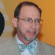cancel
Showing results for
Did you mean:New Contributor

## How much hydrogen gas and oxygen gas is needed to make one gallon of water?

How much hydrogen gas and oxygen gas is needed to make one gallon of water?  I was explaining to my nephew that a water molecule is made of hydrogen and oxygen and showed him a couple YouTube videos of a hydrogen/oxygen reaction when he posed the question. I can’t seem to find the answer via Google(I am not a chemist/scientist, my occupation is finance).  Any answers or thoughts on how to answer the question would be greatly appreciated. Thank you for your time.

2 RepliesContributor III

## Re: How much hydrogen gas and oxygen gas is needed to make one gallon of water?

Dear Eric,

That's a very good question to demonstrate the use of molar quantities in converting from one state (gaseous reactants) to another (liquid product).  If you'll allow me to use metric units instead of English ones (although the UK has gone metric for a long time itself) I will use the conversion of 1 gallon to 3.7 liters, or 3700mL.  Working backwards from the stipulated product quantity we have water mass at 1 gram/milliliter, resulting in a final mass of 3700g of water.

Now we use the molar definition to find that 3700g of water (in any form) will consist of 3700g/18.01 g/mol = 205.4 Moles of water.

The chemical equation for the combination of hydrogen and oxygen defines the ratios at which molar quantities react:

2H2 + O2 -->  2H2O

This tells us that to end up with 205.4 Moles of water, we must combine 205.4 moles of hydrogen with 102.7 moles of oxygen because the equation ratios are 2:1:2 respectively.  If you want to convert those values into either mass or volumes (at standard temperature and pressure) we can apply the appropriate conversion factors.

The mass of hydrogen required is 205.4 moles * 2g/mol = 410.8 grams.

The mass of oxygen required is 102.7 moles * 32g/mol = 3286.4 grams.

The volumes required (uncompressed) can be derived from the approximation of 22.4L/mol for ideal gases.

The volume of hydrogen required is 205.4 moles * 22.4L/mol = 4601L.

The volume of oxygen required is    102.7 moles * 22.4L/mol = 2300L.

This demonstrates the utility and necessity of using molar quantities for chemical reactions.  You can see that although the volumetric ratio of the reactants is 2:1 for hydrogen:oxygen, the mass ratio is 1:8!  We can check our calculations by adding up the calculated reactant masses and comparing them to the final product mass.  410.8g + 3286.4g = 3697.2g (only 2.8g off from our assumed mass for volume, and an overall error of just 0.07% for rounding/approximation errors).

Best regards,

Steven Cooke

Process Systems Consulting

TESC MentorNew Contributor

## Re: How much hydrogen gas and oxygen gas is needed to make one gallon of water?

Steven,

You made it extremely clear to understand and I plan on showing my nephew your answer this weekend.